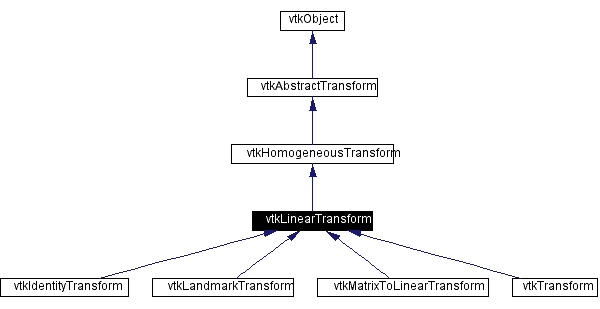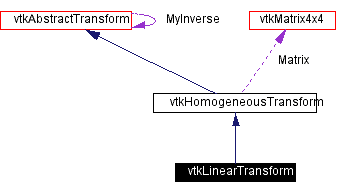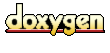Main Page   Class Hierarchy   Alphabetical List   Compound List   File List   Compound Members   File Members   Related Pages

# vtkLinearTransform Class Reference

abstract superclass for linear transformations. More...

`#include <vtkLinearTransform.h>`

Inheritance diagram for vtkLinearTransform:[legend]
Collaboration diagram for vtkLinearTransform:[legend]
List of all members.

## Public Methods

virtual const char * GetClassName ()
virtual int IsA (const char *type)
void PrintSelf (ostream &os, vtkIndent indent)
void TransformNormal (const float in, float out)
void TransformNormal (const double in, double out)
double * TransformNormal (double x, double y, double z)
double * TransformNormal (const double normal)
float * TransformFloatNormal (float x, float y, float z)
float * TransformFloatNormal (const float normal)
double * TransformDoubleNormal (double x, double y, double z)
double * TransformDoubleNormal (const double normal)
double * TransformVector (double x, double y, double z)
double * TransformVector (const double normal)
void TransformVector (const float in, float out)
void TransformVector (const double in, double out)
float * TransformFloatVector (float x, float y, float z)
float * TransformFloatVector (const float vec)
double * TransformDoubleVector (double x, double y, double z)
double * TransformDoubleVector (const double vec)
void TransformPoints (vtkPoints *inPts, vtkPoints *outPts)
virtual void TransformNormals (vtkNormals *inNms, vtkNormals *outNms)
virtual void TransformVectors (vtkVectors *inVrs, vtkVectors *outVrs)
void TransformPointsNormalsVectors (vtkPoints *inPts, vtkPoints *outPts, vtkNormals *inNms, vtkNormals *outNms, vtkVectors *inVrs, vtkVectors *outVrs)
vtkLinearTransform * GetLinearInverse ()
void InternalTransformPoint (const float in, float out)
void InternalTransformPoint (const double in, double out)
virtual void InternalTransformNormal (const float in, float out)
virtual void InternalTransformNormal (const double in, double out)
virtual void InternalTransformVector (const float in, float out)
virtual void InternalTransformVector (const double in, double out)
void InternalTransformDerivative (const float in, float out, float derivative)
void InternalTransformDerivative (const double in, double out, double derivative)

## Static Public Methods

int IsTypeOf (const char *type)
vtkLinearTransform * SafeDownCast (vtkObject *o)

## Protected Methods

vtkLinearTransform ()
~vtkLinearTransform ()
vtkLinearTransform (const vtkLinearTransform &)
void operator= (const vtkLinearTransform &)

## Detailed Description

abstract superclass for linear transformations.

Date:
2000/12/10 20:08:12
Revision:
1.21
Thanks:
Thanks to David G. Gobbi who developed this class.
vtkLinearTransform provides a generic interface for linear (affine or 12 degree-of-freedom) geometric transformations.
vtkTransform vtkIdentityTransform

Definition at line 57 of file vtkLinearTransform.h.

## Constructor & Destructor Documentation

 vtkLinearTransform::vtkLinearTransform ( ) ` [inline, protected]`
 Definition at line 191 of file vtkLinearTransform.h.

 vtkLinearTransform::~vtkLinearTransform ( ) ` [inline, protected]`
 Definition at line 192 of file vtkLinearTransform.h.

 vtkLinearTransform::vtkLinearTransform ( const vtkLinearTransform & ) ` [inline, protected]`
 Definition at line 193 of file vtkLinearTransform.h.

## Member Function Documentation

 virtual const char* vtkLinearTransform::GetClassName ( ) ` [virtual]`
 Return the class name as a string. This method is defined in all subclasses of vtkObject with the vtkTypeMacro found in vtkSetGet.h. Reimplemented from vtkHomogeneousTransform. Reimplemented in vtkIdentityTransform, vtkMatrixToLinearTransform, vtkTransform, and vtkLandmarkTransform.

 int vtkLinearTransform::IsTypeOf ( const char * type ) ` [static]`
 Return 1 if this class type is the same type of (or a subclass of) the named class. Returns 0 otherwise. This method works in combination with vtkTypeMacro found in vtkSetGet.h. Reimplemented from vtkHomogeneousTransform. Reimplemented in vtkIdentityTransform, vtkMatrixToLinearTransform, vtkTransform, and vtkLandmarkTransform.

 virtual int vtkLinearTransform::IsA ( const char * type ) ` [virtual]`
 Return 1 if this class is the same type of (or a subclass of) the named class. Returns 0 otherwise. This method works in combination with vtkTypeMacro found in vtkSetGet.h. Reimplemented from vtkHomogeneousTransform. Reimplemented in vtkIdentityTransform, vtkMatrixToLinearTransform, vtkTransform, and vtkLandmarkTransform.

 vtkLinearTransform* vtkLinearTransform::SafeDownCast ( vtkObject * o ) ` [static]`
 Will cast the supplied object to vtkObject* is this is a safe operation (i.e., a safe downcast); otherwise NULL is returned. This method is defined in all subclasses of vtkObject with the vtkTypeMacro found in vtkSetGet.h. Reimplemented from vtkHomogeneousTransform. Reimplemented in vtkIdentityTransform, vtkMatrixToLinearTransform, vtkTransform, and vtkLandmarkTransform.

 void vtkLinearTransform::PrintSelf ( ostream & os, vtkIndent indent ) ` [virtual]`
 Methods invoked by print to print information about the object including superclasses. Typically not called by the user (use Print() instead) but used in the hierarchical print process to combine the output of several classes. Reimplemented from vtkHomogeneousTransform. Reimplemented in vtkIdentityTransform, vtkMatrixToLinearTransform, vtkTransform, and vtkLandmarkTransform.

 void vtkLinearTransform::TransformNormal ( const float in, float out ) ` [inline]`
 Apply the transformation to a normal. You can use the same array to store both the input and output. Definition at line 66 of file vtkLinearTransform.h.

 void vtkLinearTransform::TransformNormal ( const double in, double out ) ` [inline]`
 Apply the transformation to a double-precision normal. You can use the same array to store both the input and output. Definition at line 71 of file vtkLinearTransform.h.

 double* vtkLinearTransform::TransformNormal ( double x, double y, double z ) ` [inline]`
 Synonymous with TransformDoubleNormal(x,y,z). Use this if you are programming in python, tcl or Java. Definition at line 76 of file vtkLinearTransform.h.

 double* vtkLinearTransform::TransformNormal ( const double normal ) ` [inline]`
 Definition at line 78 of file vtkLinearTransform.h.

 float* vtkLinearTransform::TransformFloatNormal ( float x, float y, float z ) ` [inline]`
 Apply the transformation to an (x,y,z) normal. Use this if you are programming in python, tcl or Java. Definition at line 83 of file vtkLinearTransform.h.

 float* vtkLinearTransform::TransformFloatNormal ( const float normal ) ` [inline]`
 Definition at line 89 of file vtkLinearTransform.h.

 double* vtkLinearTransform::TransformDoubleNormal ( double x, double y, double z ) ` [inline]`
 Apply the transformation to a double-precision (x,y,z) normal. Use this if you are programming in python, tcl or Java. Definition at line 94 of file vtkLinearTransform.h.

 double* vtkLinearTransform::TransformDoubleNormal ( const double normal ) ` [inline]`
 Definition at line 100 of file vtkLinearTransform.h.

 double* vtkLinearTransform::TransformVector ( double x, double y, double z ) ` [inline]`
 Synonymous with TransformDoubleVector(x,y,z). Use this if you are programming in python, tcl or Java. Definition at line 105 of file vtkLinearTransform.h.

 double* vtkLinearTransform::TransformVector ( const double normal ) ` [inline]`
 Definition at line 107 of file vtkLinearTransform.h.

 void vtkLinearTransform::TransformVector ( const float in, float out ) ` [inline]`
 Apply the transformation to a vector. You can use the same array to store both the input and output. Definition at line 112 of file vtkLinearTransform.h.

 void vtkLinearTransform::TransformVector ( const double in, double out ) ` [inline]`
 Apply the transformation to a double-precision vector. You can use the same array to store both the input and output. Definition at line 117 of file vtkLinearTransform.h.

 float* vtkLinearTransform::TransformFloatVector ( float x, float y, float z ) ` [inline]`
 Apply the transformation to an (x,y,z) vector. Use this if you are programming in python, tcl or Java. Definition at line 122 of file vtkLinearTransform.h.

 float* vtkLinearTransform::TransformFloatVector ( const float vec ) ` [inline]`
 Definition at line 128 of file vtkLinearTransform.h.

 double* vtkLinearTransform::TransformDoubleVector ( double x, double y, double z ) ` [inline]`
 Apply the transformation to a double-precision (x,y,z) vector. Use this if you are programming in python, tcl or Java. Definition at line 133 of file vtkLinearTransform.h.

 double* vtkLinearTransform::TransformDoubleVector ( const double vec ) ` [inline]`
 Definition at line 139 of file vtkLinearTransform.h.

 void vtkLinearTransform::TransformPoints ( vtkPoints * inPts, vtkPoints * outPts ) ` [virtual]`
 Apply the transformation to a series of points, and append the results to outPts. Reimplemented from vtkHomogeneousTransform. Reimplemented in vtkIdentityTransform. Referenced by vtkTransform::MultiplyPoints().

 virtual void vtkLinearTransform::TransformNormals ( vtkNormals * inNms, vtkNormals * outNms ) ` [virtual]`
 Apply the transformation to a series of normals, and append the results to outNms. Reimplemented in vtkIdentityTransform. Referenced by vtkTransform::MultiplyNormals().

 virtual void vtkLinearTransform::TransformVectors ( vtkVectors * inVrs, vtkVectors * outVrs ) ` [virtual]`
 Apply the transformation to a series of vectors, and append the results to outVrs. Reimplemented in vtkIdentityTransform. Referenced by vtkTransform::MultiplyVectors().

 void vtkLinearTransform::TransformPointsNormalsVectors ( vtkPoints * inPts, vtkPoints * outPts, vtkNormals * inNms, vtkNormals * outNms, vtkVectors * inVrs, vtkVectors * outVrs ) ` [virtual]`
 Apply the transformation to a combination of points, normals and vectors. Reimplemented from vtkHomogeneousTransform. Reimplemented in vtkIdentityTransform.

 vtkLinearTransform* vtkLinearTransform::GetLinearInverse ( ) ` [inline]`
 Just like GetInverse, but it includes a typecast to vtkLinearTransform. Definition at line 165 of file vtkLinearTransform.h.

 void vtkLinearTransform::InternalTransformPoint ( const float in, float out ) ` [virtual]`
 This will calculate the transformation without calling Update. Meant for use only within other VTK classes. Reimplemented from vtkHomogeneousTransform. Reimplemented in vtkIdentityTransform.

 void vtkLinearTransform::InternalTransformPoint ( const double in, double out ) ` [virtual]`
 Reimplemented from vtkHomogeneousTransform. Reimplemented in vtkIdentityTransform.

 virtual void vtkLinearTransform::InternalTransformNormal ( const float in, float out ) ` [virtual]`
 This will calculate the transformation without calling Update. Meant for use only within other VTK classes. Reimplemented in vtkIdentityTransform.

 virtual void vtkLinearTransform::InternalTransformNormal ( const double in, double out ) ` [virtual]`
 Reimplemented in vtkIdentityTransform.

 virtual void vtkLinearTransform::InternalTransformVector ( const float in, float out ) ` [virtual]`
 This will calculate the transformation without calling Update. Meant for use only within other VTK classes. Reimplemented in vtkIdentityTransform.

 virtual void vtkLinearTransform::InternalTransformVector ( const double in, double out ) ` [virtual]`
 Reimplemented in vtkIdentityTransform.

 void vtkLinearTransform::InternalTransformDerivative ( const float in, float out, float derivative ) ` [virtual]`
 This will calculate the transformation as well as its derivative without calling Update. Meant for use only within other VTK classes. Reimplemented from vtkHomogeneousTransform. Reimplemented in vtkIdentityTransform.

 void vtkLinearTransform::InternalTransformDerivative ( const double in, double out, double derivative ) ` [virtual]`
 Reimplemented from vtkHomogeneousTransform. Reimplemented in vtkIdentityTransform.

 void vtkLinearTransform::operator= ( const vtkLinearTransform & ) ` [inline, protected]`
 Definition at line 194 of file vtkLinearTransform.h.

The documentation for this class was generated from the following file:

Generated on Wed Nov 21 12:52:45 2001 for VTK by1.2.11.1 written by Dimitri van Heesch, © 1997-2001